# Lines And angles worksheet for Class 7 Maths

Question 1
Fill in the blanks:
a) Two ______________ are said to form linear pair of the angels if their non common arms are two opposite rays.
b) if a ray stands on a line then the sum of the adjacent angles so formed is _______________.
c) The sum of all angles around a point is _________________.
d) An angle which is equal to its complement is _____________.
e) Two angles are called a pair of _____________________ if their arms form two pairs of opposite rays.
f) If two lines intersect then the Vertically opposite angles are  _____________
g) A line which intersects two or more lines at distinct points is called a ___________________
h) You can draw _____________ transversals on a line.
(i) If  two lines are intersected by a transversal such that any pair of corresponding angles are equal then the lines are _________________
Question 2
Two parallel lines I and m are intersected by a transversal t. If the interior angles on same side of transversal are $(2x- 8)^o$ and $(3x-7)^o$  Find the measure of these angles.
Question 3
In the given fig. I is parallel to m and p ll q. Find the measure of each of the angles a,b,c,d.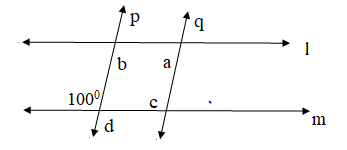Question 4
In the given figure below. AB II CD and AD is produced to E so that $\angle BAE =125^o$ . if $\angle ABC =x^o$ , $\angle BCD =y^o$  and $\angle CDE =z^o$ and $\angle ADC =x^o$ Find the values of x,y and z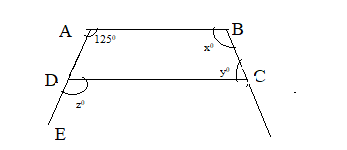Question 5
In the given below figure rays OA,OB,OC and OD intersect at a point. Find the value of x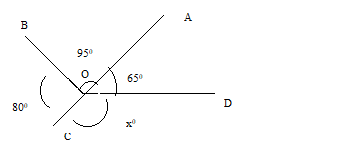Question 6
True and False statement
a. Sum of two complementary angles is 180°.
b.Sum of two supplementary angles is 180°.
c. Sum of interior angles on the same side of a transversal with two parallel lines is 90°.
d. Vertically opposite angles are equal.
e. A linear pair may have two acute angles
f. Two supplementary angles are always obtuse angles
Question 7
In below figure , AB||CD, AF||ED,  $\angle AFC = 68^o$ and $\angle FED = 42^o$ Find $\angle EFD$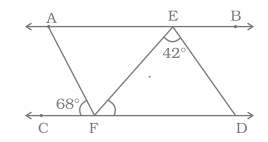Question 8
In below figure, l ||m ||n. ∠ QPS = 35° and ∠ QRT = 55°. Find ∠PQR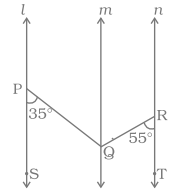1.
b. 180
c. 360
d.45
e. Vertically opposite angles
f. equal
g. transversal
h. 0
i. parallel
2. 70 ,110
3. a=80 ,b=100,c=100,d=100
4. x=65 ,y =125 ,z=125
5. 120
6.
a. false
b. true
c. false
d. true
e. false
f. false
7. 70
8. 90